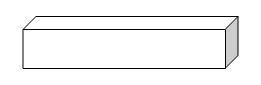# 3-D Shapes and Algebra

3-D Shapes

While children will be expected to know a lot about the properties of 2-D shapes (squares, triangles and the like), there is little needed to be known about the properties of 3-D shapes. Certainly your child will need to understand about prisms (those shapes which are the same all the way through their length) but others will be explained if they are used in SATs or entrance papers. It is not generally expected for anyone to be able to recall the surface area of a sphere or the volume of a cone, for example.

The surface area of a cube or cuboid shape:The above shape is a cuboid. If you slice it at any point of its length it will produce the same shape, so it is a prism. The surface area is the sum of the areas of the six sides. Remember, area is given in square cm / m or whatever unit you are using.

The surface area of a cube, given that the sides are all the same, is six lots of the area of one side.

The volume of a prism:

Whatever the shape of the prism (cuboid, triangular prism, cylinder etc) the best way of calculating the volume is to establish the area of the end shape and multiply that by the length of the prism. For example, a triangular prism with a triangle of area 10cm2 and length 9cm, will have a volume of (10cm2 x 9cm) 90cm3. Note that the units are CUBED and not just SQUARED. Children constantly make this error, putting cm2 instead of cm3, and therefore getting a wrong answer. Such tiny details really matter!

Algebra

Children have to understand the basics of algebra as they sit entrance papers and SATs; don't worry though, the whole process is much easier than many people remember from school! Sometimes a question will be presented as a problem which leads to the algebraic equation while other times it is just set out as an equation from the beginning.

Let's look at this example:

If there are x books in the school library and there are 12 shelves with the same amount of books on each, how many books are there on each shelf?

The first thing to do is not panic because a letter is used. It simply means 'a number that we don't know' and we're allowed to present an answer in terms of x or whatever the question-setters have chosen to use. As long as you expect to find a specific answer, you'll get yourself confused.

The easiest way to understand the question is to think in terms of a specific number and see what the process would be. Then you can take the idea and explain it in terms of x.

For example, if the total number of books was 120 and the number of shelves was 12, the number of books per shelf would be ( 120 ÷ 12 ) = 10.

Now, we have established a process to work out the answer. We simply have to replace our suggested 120 with the term 'x'. It means that our answer is

x ÷ 12 or x⁄12

People who get lost with algebra are those who look to go beyond this. There is no number that answers the question - as we have no idea what x is, we simply leave it like that. In a way, it's just about being able to show the sum that we have to do to reach an answer; whatever the value of x is, we have a sum that we could use to get the relevant answer.